## Selina Concise Mathematics Class 6 ICSE Solutions Chapter 18 Fundamental Concepts

Selina Publishers Concise Mathematics Class 6 ICSE Solutions Chapter 18 Fundamental Concepts

APlusTopper.com provides step by step solutions for Selina Concise ICSE Solutions for Class 6 Mathematics. You can download the Selina Concise Mathematics ICSE Solutions for Class 6 with Free PDF download option. Selina Publishers Concise Mathematics for Class 6 ICSE Solutions all questions are solved and explained by expert mathematic teachers as per ICSE board guidelines.

IMPORTANT POINTS

1. Algebra : Algebra is a generalized form of Arithmetic. In Arithmetic, we use numbers, such as : 3, – 8, 0.63, etc., each of which has one definite value ; whereas in Algebra, we use letters along with numbers.
For Example : 5x, 3x – 4, 7a + b, 3y – 5x, x + 3y – 9z, etc.
The letters used in Algebra are called variables or literal numbers or simply literals.
2. Signs and Symbols : In Algebra, the signs +, -, x and ÷ are used with the same meaning as in Arithmetic.
Following sign and symbols are frequently used in algebra and have the same meanings as they have in any other branch of Mathematics.
= means, “is equal to”
≠ means, “is not equal to”
< means, “is less than” > means, “is greater than”
$$\nless$$ means, “is not less than”
$$\ngtr$$ means, “is not greater than”
∴ means, “therefore”
∵ means, “because” or “since”
~ means, “difference between”
⇒ means, “implies that”.
3. To Write a Given Statement in Algebraic Form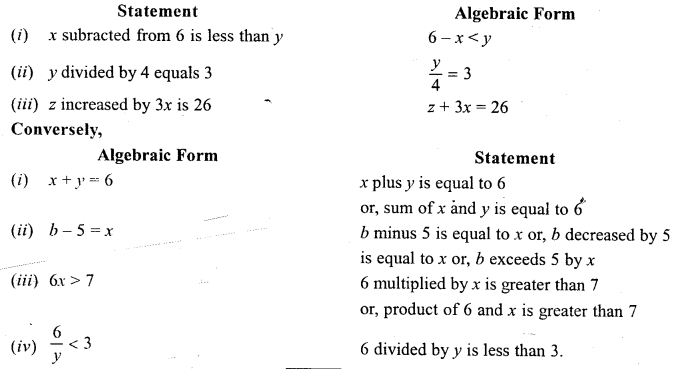### Fundamental Concepts Exercise 18A – Selina Concise Mathematics Class 6 ICSE Solutions

Question 1.
Express each of the following statements in algebraic form :
(i) The sum of 8 and x is equal to y.
(ii) x decreased by 5 is equal to y.
(iii) The sum of 2 and x is greater than y.
(iv) The sum of x and y is less than 24.
(v) 15 multiplied by m gives 3n.
(vi) Product of 8 and y is equal to 3x.
(vii) 30 divided by b is equal to p.
(viii) z decreased by 3x is equal to y.
(ix) 12 times of x is equal to 5z.
(x) 12 times of x is greater than 5z.
(xi) 12 times of x is less than 5z.
(xii) 3z subtracted from 45 is equal to y.
(xiii) 8x divided by y is equal to 2z.
(xiv) 7y subtracted from 5x gives 8z.
(xv) 7y decreased by 5x gives 8z.
Solution: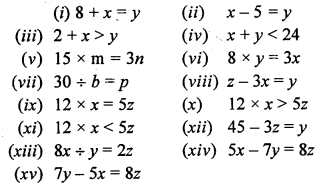Question 2.
For each of the following algebraic expressions, write a suitable statement in words:
(i) 3x + 8=15
(ii) 7 – y > x
(iii) 2y – x < 12
(iv) 5 ÷ z = 5
(v) a + 2b > 18
(vi) 2x – 3y= 16
(vii) 3a – 4b > 14
(viii) b + 7a < 21
(ix) (16 + 2a) – x > 25
(x) (3x + 12) – y < 3a
Solution:
(i) 3x plus 8 is equal to 15
(ii) 1 decreased by y is greater than x
(iii) 2y decreased by x is less than 12
(iv) 5 divided by z is equal to 5
(v) a increased by 2b is greater than 18
(vi) 2x decreased by 3y is equal to 16
(vii) 3a decreased by 4b is greater than 14
(viii) b increased la is less than 21
(ix) The sum of 16 and 2a decreased by x is greater than 25
(x) The sum of 3x and 12 decreased by y is less than 3a.

### Fundamental Concepts Exercise 18B – Selina Concise Mathematics Class 6 ICSE Solutions

Question 1.
Separate the constants and the variables from each of the following: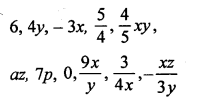Solution: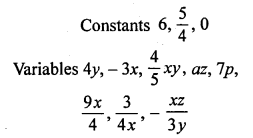Question 2.
Group the like terms together :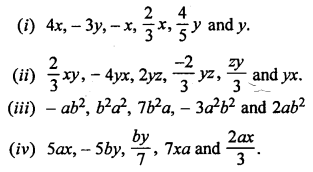Solution: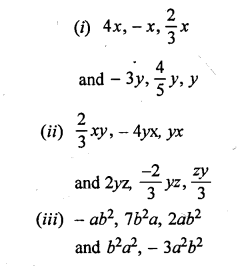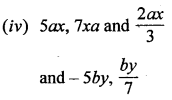Question 3.
State whether true or false :
(i) 16 is a constant and y is a variable but 16y is variable.
(ii) 5x has two terms 5 and x.
(iii) The expression 5 + x has two terms 5 and x
(iv) The expression 2x2 + x is a trinomial.
(v) ax2 + bx + c is a trinomial.
(vi) 8 x ab is a binomial.
(vii) 8 + ab is a binomial.
(viii) x3 – 5xy + 6x + 7 is a polynomial.
(ix) x3 – 5xy + 6x + 7 is a multinomial.
(x) The coefficient of x in 5x is 5x.
(xi) The coefficient of ab in – ab is – 1.
(xii) The coefficient of y in – 3xy is – 3
Solution:
(i) True
(ii) False
(iii) True
(iv) False
(v) True
(vi) False
(vii) True
(viii) True
(ix) True
(x) False
(xi) True
(xii) False

Question 4.
State the number of terms in each of the following expressions :Solution:
(i) 2 terms
(ii) 2 terms
(iii) 2 terms
(iv) 2 terms
(v) 3 terms
(vi) 2 term
(vii) 2 terms
(viii) 3 terms
(ix) 3 terms

Question 5.
State whether true or false:
(i) xy and – yx are like terms.
(ii) x2y and – y2x are like terms.
(iii) a and – a are like terms.
(iv) – ba and 2ab are unlike terms.
(v) 5 and 5x are like terms.
(vi) 3xy and 4xyz are unlike terms.
Solution:
(i) True
(ii) False
(iii) True
(iv) False
(v) False
(vi) True

Question 6.
For each expression, given below, state whether it is a monomial, or a binomial or a trinomial.Solution:
(i) Monomial
(ii) Binomial
(iii) Monomial
(iv) Monomial
(v) Trinomial
(vi) Binomial
(vii) Trinomial
(viii) Binomial
(ix) Trinomial

Question 7.
Write down the coefficient of x in the following monomial :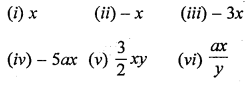Solution:Question 8.
Write the coefficient of :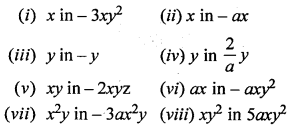Solution: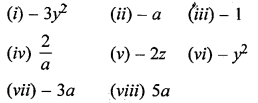Question 9.
State the numeral coefficient of the following monomials :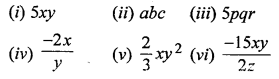(vii) – 7x ÷ y
(viii) – 3x ÷ (2y)
Solution:Question 10.
Write the degree of each of the following polynomials :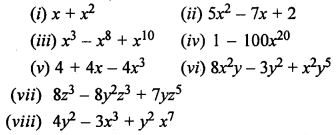Solution:
(i) 2
(ii) 2
(iii) 10
(iv) 20
(v) 3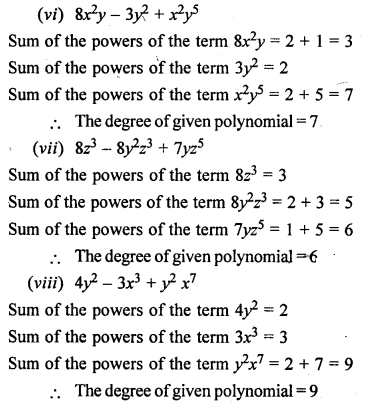### Fundamental Concepts Revision Exercise – Selina Concise Mathematics Class 6 ICSE Solutions

Question 1.
Express each of the following statements in algebraic form :
(i) The sum of 3x and 4y is 8.
(ii) 5x decreased by 7 gives y.
(iii) 31 added to 4x gives 6x.
(iv) 3x subtracted from 89 gives 44.
Solution:
(i) 3x + 4y = 8
(ii) 5x – 7 = y
(iii) 4x + 37 = 6x
(iv) 89 – 3x = 44

Question 2.
Group the like terms :Solution: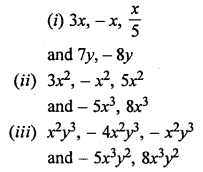Question 3.
Write the number of terms in each of the following polynomials :Solution:
(i) 2 terms
(ii) 3 terms
(iii) 3 terms
(iv) 4 terms
(v) 3 terms

Question 4.
For each expression, given below, state whether it is a monomial, or a binomial or a trinomial:
(i) x + y
(ii) 5x – 4y
(iii) 7x2 + 5x + 8
(iv) 64 + 3 ÷ 6
(v) 9 ÷ a x b
(vi) 8a ÷ b
Solution:
(i) binomial
(ii) binomial
(iii) trinomial
(iv) 6a + 3 ÷ b = 6a + $$\frac { 3 }{ b }$$
It has two terms
It is binomial
(v) 9 ÷ a x b = $$\frac { 9b }{ a }$$
It has one term
It is monomial.
(vi) monomial

Question 5.
Write the coefficient of x2y in :
(i) -7x2yz
(ii) 8abx2y
(iii) – x2y
Solution:
(i) – 7z
(ii) 8ab
(iii) -1

Question 6.
Write the coefficient of :
(i) x2 in – 8x2y
(ii) y in -4y
(iii) x in – xy2
Solution:
(i) – 8y
(ii) – 4
(iii) – y2

Question 7.
Write the numeral coefficient in :Solution:Question 8.
Write the degree of each of the following polynomials :Solution:
(i) 8
(ii) 4
(iii) 2
(iv) 1
(v) 3Question 9.
Write each statement, given below in algebraic form :
(i) 28 more than twice of x is equal to 45.
(ii) 3y reduced by 5z is greater than 8x.
(iii) 6x divided by 13y is less than 17.
(iv) 9 multiplied by 5x is equal to 2y.
Solution: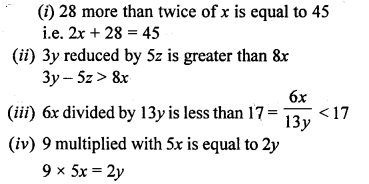Question 10.
State whether true or false :
(i) If 23 is a constant and x is a variable, 23 + x is constant.
(ii) If 23 is a constant and x is a variable, 23x is a variable.
(iii) If y is a variable and 57 is a constant, y – 57 is a variable.
(iv) If 3x and 2y are variable; each of 3x + 2y, 3x – 2y, 3x ÷ 2y and 3x x 2y is a variable.
Solution:
(i) False
Sum of a constant and a variable is also variable.
(ii) True
Product of a constant and a variable is variable.
(iii) True
Constant subtracted from a variable is also variable.
(iv) True
Sum, difference product or quotient of two variables is also variable.